1595358000

# Design a simple neural network with numpy

“Tell me and I forget, teach me and I may remember, involve me and I learn.” — Benjamin Franklin

I have often heard people around me saying that Neural Networks are a difficult concept to grasp and guess what! I also thought of it as the same before I got my hands dirty and dived into the NN World! So here is a piece that I hope will surely help you understand what Neural Networks are. Happy Reading!

This is one of the best things that I have tried while learning about neural networks and this helped me to understand Deep Neural Networks a lot better. I am extremely psyched to share this entire process with everyone. So without any further ado, let’s begin !!

First of all, I would like to go through a few basic terms that we will be requiring to work with this:

Neural Networks: Broadly speaking, Neural networks are a set of algorithms, modeled loosely after the human brain, that is designed to recognize patterns. They interpret sensory data through a kind of machine perception, labeling, or clustering raw input. So I guess I won’t be wrong if I say that we can do a job better if we repeatedly to it for a number of times, can’t we? How many times have you tried before, before you have perfected the amount of salt in your food?

Numpy: Numpy is the fundamental package for coding in python and performing scientific operations. We will be taking a lot of help from this package while designing the simplistic NN. To import NumPy into your Python notebook (I prefer to use Jupyter notebook, you may use Google Colab, by which you can take advantage of Google’s state of the art cloud servers as well as their CPUs, GPUs, and TPUs.) Here’s a link to Colab Notebook:

Inputs: These will be the data we will be providing the model to train on. For this exercise, we will choose inputs from NumPy’s uniform distribution, where given the initial and final values, NumPy will create an array for you, where each element had the equal probability of being chosen from the specified interval. This can be any other distribution and is absolutely random, readers can try with normal distribution on their own.

Targets: These will be the desired values for each of the inputs. Here we will take a random equation, and generate the targets manually, on our own. However, this will **not be the case **in real life. This example is only chosen to demonstrate how neural networks work. These are denoted by tᵢ.

Outputs: These will be the values that the machine will predict based on the inputs. The difference between targets and the outputs will be denoted by the variable **deltas **later on in the coding part. These are denoted by yᵢ.

Loss Function: Sometimes this is also referred to as the cost function. Our target will be to minimize this loss. The Loss Function in our context evaluates the accuracy of the outputs, regarding the targets. In this example, we will be using the L2-Norm Loss Function. It is given by :

L(y,t) = ∑(yᵢ-tᵢ)²

Optimization Algorithm: This algorithm consists of mechanics through which we may vary the parameters(weights and biases) to optimize the Loss function. Here we will be using the n-parameter gradient descent. Without going too deep into mathematics,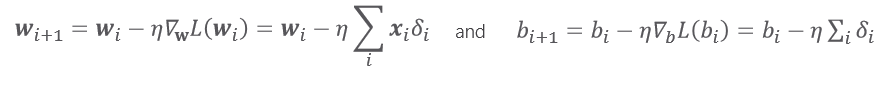where the wᵢ represents the weights and the bᵢ represents the biases for each input variables and η is the learning rate of the model.

If anyone of you reading this is uncomfortable with matrix multiplication, I suggest you read this.

Now let’s get our hands dirty and begin coding !!

The only library we will be needing for this will be NumPy.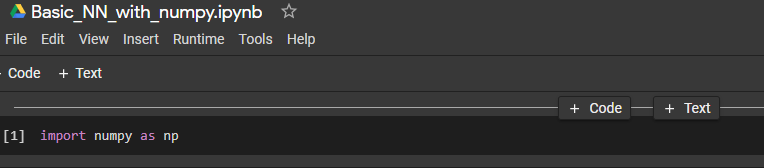Let me call the variables x and z. As I said in the inputs section, I will generate the inputs from the NumPy random uniform distribution. I will also put them in a matrix form using the NumPy column stack method. The dimension of this input matrix is (1000 X 2), where 1000 is the number of observations and 2 is the number of input variables.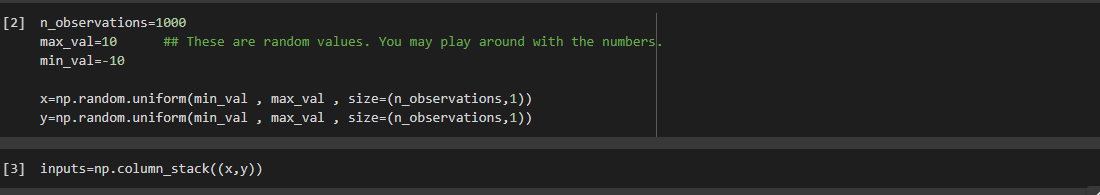Now I will be generating the targets. The Targets will be generated by the random equation in the following code. Our model’s main aim will be to determine the weights(the coefficients) and the bias(the constant). To randomize our data a bit, I will be adding noise to it.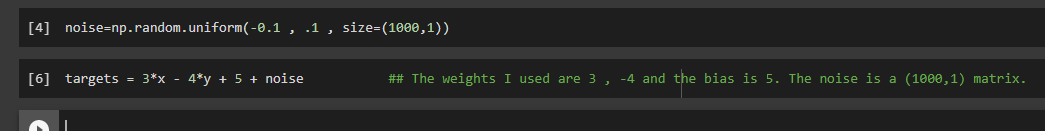Beware: Do not add a very high noise as it will destroy the underlying trend of the data.

In this way, I have generated a fake data that we will use to feed our model with. The only thing I have been left with is to initialize the weights and the bias. Again, I will be using the NumPy random uniform distribution.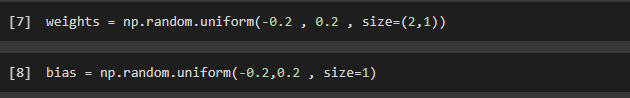Now is the tricky part where I will be designing the network.

For training the network, I will be using 100 iterations. Here I am setting η(learning_rate) to be 0.01. We do not want this value to be too high. For this part, you will be requiring very basic python coding skills.

#numpy-array #machine-learning #matplotlib #numpy #neural-networks

## Buddha Community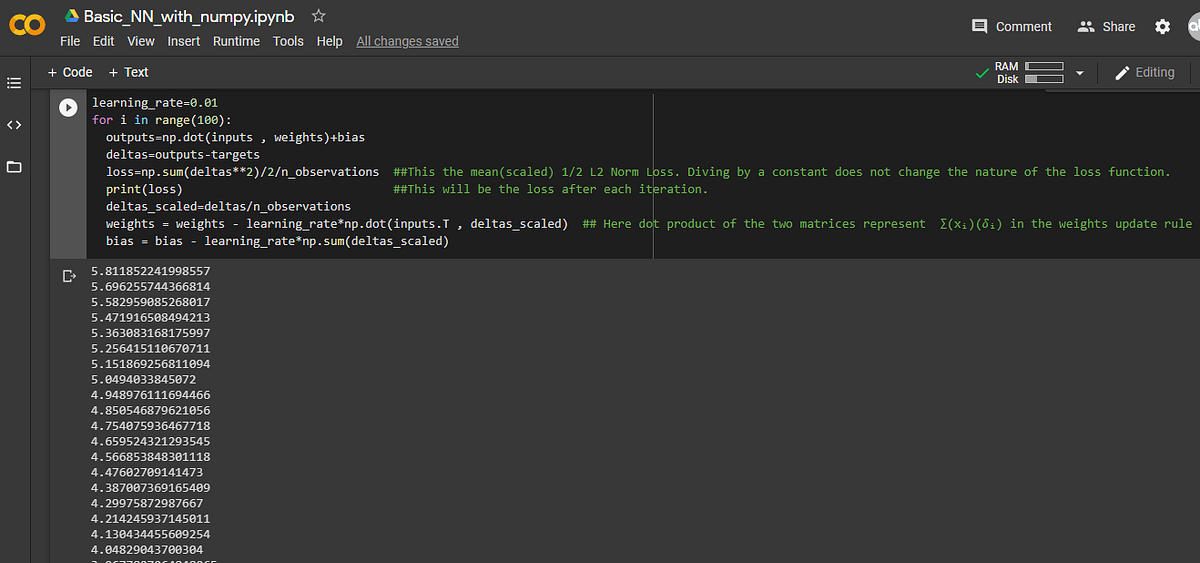1595358000

## Design a simple neural network with numpy

“Tell me and I forget, teach me and I may remember, involve me and I learn.” — Benjamin Franklin

I have often heard people around me saying that Neural Networks are a difficult concept to grasp and guess what! I also thought of it as the same before I got my hands dirty and dived into the NN World! So here is a piece that I hope will surely help you understand what Neural Networks are. Happy Reading!

This is one of the best things that I have tried while learning about neural networks and this helped me to understand Deep Neural Networks a lot better. I am extremely psyched to share this entire process with everyone. So without any further ado, let’s begin !!

First of all, I would like to go through a few basic terms that we will be requiring to work with this:

Neural Networks: Broadly speaking, Neural networks are a set of algorithms, modeled loosely after the human brain, that is designed to recognize patterns. They interpret sensory data through a kind of machine perception, labeling, or clustering raw input. So I guess I won’t be wrong if I say that we can do a job better if we repeatedly to it for a number of times, can’t we? How many times have you tried before, before you have perfected the amount of salt in your food?

Numpy: Numpy is the fundamental package for coding in python and performing scientific operations. We will be taking a lot of help from this package while designing the simplistic NN. To import NumPy into your Python notebook (I prefer to use Jupyter notebook, you may use Google Colab, by which you can take advantage of Google’s state of the art cloud servers as well as their CPUs, GPUs, and TPUs.) Here’s a link to Colab Notebook:

Inputs: These will be the data we will be providing the model to train on. For this exercise, we will choose inputs from NumPy’s uniform distribution, where given the initial and final values, NumPy will create an array for you, where each element had the equal probability of being chosen from the specified interval. This can be any other distribution and is absolutely random, readers can try with normal distribution on their own.

Targets: These will be the desired values for each of the inputs. Here we will take a random equation, and generate the targets manually, on our own. However, this will **not be the case **in real life. This example is only chosen to demonstrate how neural networks work. These are denoted by tᵢ.

Outputs: These will be the values that the machine will predict based on the inputs. The difference between targets and the outputs will be denoted by the variable **deltas **later on in the coding part. These are denoted by yᵢ.

Loss Function: Sometimes this is also referred to as the cost function. Our target will be to minimize this loss. The Loss Function in our context evaluates the accuracy of the outputs, regarding the targets. In this example, we will be using the L2-Norm Loss Function. It is given by :

L(y,t) = ∑(yᵢ-tᵢ)²

Optimization Algorithm: This algorithm consists of mechanics through which we may vary the parameters(weights and biases) to optimize the Loss function. Here we will be using the n-parameter gradient descent. Without going too deep into mathematics,where the wᵢ represents the weights and the bᵢ represents the biases for each input variables and η is the learning rate of the model.

If anyone of you reading this is uncomfortable with matrix multiplication, I suggest you read this.

Now let’s get our hands dirty and begin coding !!

The only library we will be needing for this will be NumPy.Let me call the variables x and z. As I said in the inputs section, I will generate the inputs from the NumPy random uniform distribution. I will also put them in a matrix form using the NumPy column stack method. The dimension of this input matrix is (1000 X 2), where 1000 is the number of observations and 2 is the number of input variables.Now I will be generating the targets. The Targets will be generated by the random equation in the following code. Our model’s main aim will be to determine the weights(the coefficients) and the bias(the constant). To randomize our data a bit, I will be adding noise to it.Beware: Do not add a very high noise as it will destroy the underlying trend of the data.

In this way, I have generated a fake data that we will use to feed our model with. The only thing I have been left with is to initialize the weights and the bias. Again, I will be using the NumPy random uniform distribution.Now is the tricky part where I will be designing the network.

For training the network, I will be using 100 iterations. Here I am setting η(learning_rate) to be 0.01. We do not want this value to be too high. For this part, you will be requiring very basic python coding skills.

#numpy-array #machine-learning #matplotlib #numpy #neural-networks1609840501

## Landscapes Website Design | Nature Landscapes Website Designer

Most landscapers think of their website as an online brochure. In reality of consumers have admitted to judging a company’s credibility based on their web design, making your website a virtual sales rep capable of generating massive amounts of leads and sales. If your website isn’t actively increasing leads and new landscaping contracts, it may be time for a redesign.

DataIT Solutions specializes in landscape website designing that are not only beautiful but also rank well in search engine results and convert your visitors into customers. We’ve specialized in the landscaping industry for over 10 years, and we look at your business from an owner’s perspective.

Why use our Landscapes for your landscape design?

• Superior experience
• Friendly personal service
• Choice of design layout
• Budget sensitive designs
• Impartial product choice and advice
• Planting and lighting designs

If you are a gardener or have a gardening company please do not hesitate to contact us for a quote.
Need help with your website?
Get in touch

#nature landscapes website design #landscapes website design #website design #website designing #website designer #designer1623135499

## No Code introduction to Neural Networks

### The simple architecture explained

Neural networks have been around for a long time, being developed in the 1960s as a way to simulate neural activity for the development of artificial intelligence systems. However, since then they have developed into a useful analytical tool often used in replace of, or in conjunction with, standard statistical models such as regression or classification as they can be used to predict or more a specific output. The main difference, and advantage, in this regard is that neural networks make no initial assumptions as to the form of the relationship or distribution that underlies the data, meaning they can be more flexible and capture non-standard and non-linear relationships between input and output variables, making them incredibly valuable in todays data rich environment.

In this sense, their use has took over the past decade or so, with the fall in costs and increase in ability of general computing power, the rise of large datasets allowing these models to be trained, and the development of frameworks such as TensforFlow and Keras that have allowed people with sufficient hardware (in some cases this is no longer even an requirement through cloud computing), the correct data and an understanding of a given coding language to implement them. This article therefore seeks to be provide a no code introduction to their architecture and how they work so that their implementation and benefits can be better understood.

Firstly, the way these models work is that there is an input layer, one or more hidden layers and an output layer, each of which are connected by layers of synaptic weights¹. The input layer (X) is used to take in scaled values of the input, usually within a standardised range of 0–1. The hidden layers (Z) are then used to define the relationship between the input and output using weights and activation functions. The output layer (Y) then transforms the results from the hidden layers into the predicted values, often also scaled to be within 0–1. The synaptic weights (W) connecting these layers are used in model training to determine the weights assigned to each input and prediction in order to get the best model fit. Visually, this is represented as:

#machine-learning #python #neural-networks #tensorflow #neural-network-algorithm #no code introduction to neural networks1595235180

## NumPy Features - Why we should use Numpy?

Welcome to DataFlair!!! In this tutorial, we will learn Numpy Features and its importance.

NumPy is a library for the Python programming language, adding support for large, multi-dimensional arrays and matrices, along with a large collection of high-level mathematical functions to operate on these arrays

NumPy (Numerical Python) is an open-source core Python library for scientific computations. It is a general-purpose array and matrices processing package. Python is slower as compared to Fortran and other languages to perform looping. To overcome this we use NumPy that converts monotonous code into the compiled form.#### NumPy Features

These are the important features of NumPy:

#### 1. High-performance N-dimensional array object

This is the most important feature of the NumPy library. It is the homogeneous array object. We perform all the operations on the array elements. The arrays in NumPy can be one dimensional or multidimensional.

#### a. One dimensional array

The one-dimensional array is an array consisting of a single row or column. The elements of the array are of homogeneous nature.

#### b. Multidimensional array

In this case, we have various rows and columns. We consider each column as a dimension. The structure is similar to an excel sheet. The elements are homogenous.

#### 2. It contains tools for integrating code from C/C++ and Fortran

We can use the functions in NumPy to work with code written in other languages. We can hence integrate the functionalities available in various programming languages. This helps implement inter-platform functions.

#numpy tutorials #features of numpy #numpy features #why use numpy #numpy1595235240

## NumPy Applications - Uses of Numpy

In this Numpy tutorial, we will learn Numpy applications.

NumPy is a basic level external library in Python used for complex mathematical operations. NumPy overcomes slower executions with the use of multi-dimensional array objects. It has built-in functions for manipulating arrays. We can convert different algorithms to can into functions for applying on arrays.NumPy has applications that are not only limited to itself. It is a very diverse library and has a wide range of applications in other sectors. Numpy can be put to use along with Data Science, Data Analysis and Machine Learning. It is also a base for other python libraries. These libraries use the functionalities in NumPy to increase their capabilities.#### 1. An alternative for lists and arrays in Python

Arrays in Numpy are equivalent to lists in python. Like lists in python, the Numpy arrays are homogenous sets of elements. The most important feature of NumPy arrays is they are homogenous in nature. This differentiates them from python arrays. It maintains uniformity for mathematical operations that would not be possible with heterogeneous elements. Another benefit of using NumPy arrays is there are a large number of functions that are applicable to these arrays. These functions could not be performed when applied to python arrays due to their heterogeneous nature.

#### 2. NumPy maintains minimal memory

Arrays in NumPy are objects. Python deletes and creates these objects continually, as per the requirements. Hence, the memory allocation is less as compared to Python lists. NumPy has features to avoid memory wastage in the data buffer. It consists of functions like copies, view, and indexing that helps in saving a lot of memory. Indexing helps to return the view of the original array, that implements reuse of the data. It also specifies the data type of the elements which leads to code optimization.

#### 3. Using NumPy for multi-dimensional arrays

We can also create multi-dimensional arrays in NumPy.These arrays have multiple rows and columns. These arrays have more than one column that makes these multi-dimensional. Multi-dimensional array implements the creation of matrices. These matrices are easy to work with. With the use of matrices the code also becomes memory efficient. We have a matrix module to perform various operations on these matrices.

#### 4. Mathematical operations with NumPy

Working with NumPy also includes easy to use functions for mathematical computations on the array data set. We have many modules for performing basic and special mathematical functions in NumPy. There are functions for Linear Algebra, bitwise operations, Fourier transform, arithmetic operations, string operations, etc.

#numpy tutorials #applications of numpy #numpy applications #uses of numpy #numpy